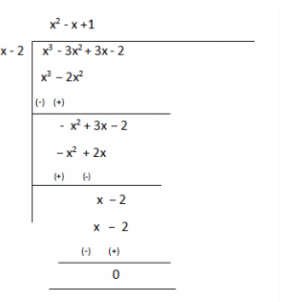Guru

# On dividing x3-3×2+x+2 by a polynomial g(x), the quotient and remainder were x–2 and –2x+4, respectively. Find g(x). Q.4

• 0

The important question of class 10th. I don’t know how to solve this question. Please help me for solving this question. Because it is very tough question. On dividing x3-3×2+x+2 by a polynomial g(x), the quotient and remainder were x–2 and –2x+4, respectively. Find g(x).

Share

1. Given,

Dividend, p(x) = x3-3x2+x+2

Quotient = x-2

Remainder = –2x+4

We have to find the value of Divisor, g(x) =?

As we know,

Dividend = Divisor × Quotient + Remainder

∴ x3-3x2+x+2 = g(x)×(x-2) + (-2x+4)

x3-3x2+x+2-(-2x+4) = g(x)×(x-2)

Therefore, g(x) × (x-2) = x3-3x2+x+2

Now, for finding g(x) we will divide x3-3x2+x+2 with (x-2)Therefore, g(x) = (x2–x+1)

• 0